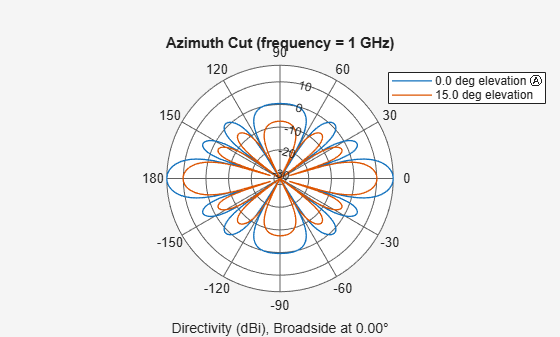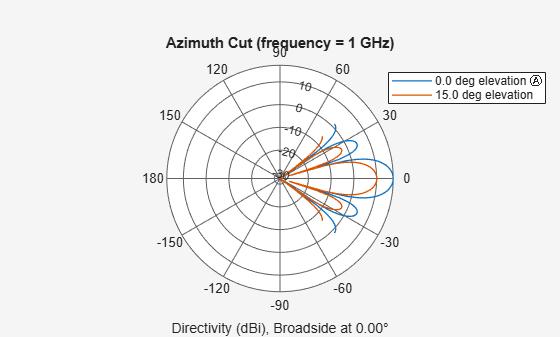# patternAzimuth

System object: phased.URA
Package: phased

Plot URA array directivity or pattern versus azimuth

## Syntax

```patternAzimuth(sArray,FREQ) patternAzimuth(sArray,FREQ,EL) patternAzimuth(sArray,FREQ,EL,Name,Value) PAT = patternAzimuth(___) ```

## Description

`patternAzimuth(sArray,FREQ)` plots the 2-D array directivity pattern versus azimuth (in dBi) for the array `sArray` at zero degrees elevation angle. The argument `FREQ` specifies the operating frequency.

The integration used when computing array directivity has a minimum sampling grid of 0.1 degrees. If an array pattern has a beamwidth smaller than this, the directivity value will be inaccurate.

`patternAzimuth(sArray,FREQ,EL)`, in addition, plots the 2-D array directivity pattern versus azimuth (in dBi) for the array `sArray` at the elevation angle specified by `EL`. When `EL` is a vector, multiple overlaid plots are created.

`patternAzimuth(sArray,FREQ,EL,Name,Value)` plots the array pattern with additional options specified by one or more `Name,Value` pair arguments.

`PAT = patternAzimuth(___)` returns the array pattern. `PAT` is a matrix whose entries represent the pattern at corresponding sampling points specified by the `'Azimuth'` parameter and the `EL` input argument.

## Input Arguments

expand all

Uniform rectangular array, specified as a `phased.URA` System object.

Example: `sArray= phased.URA;`

Frequency for computing directivity and pattern, specified as a positive scalar. Frequency units are in hertz.

• For an antenna or microphone element, `FREQ` must lie within the range of values specified by the `FrequencyRange` or the `FrequencyVector` property of the element. Otherwise, the element produces no response and the directivity is returned as `–Inf`. Most elements use the `FrequencyRange` property except for `phased.CustomAntennaElement` and `phased.CustomMicrophoneElement`, which use the `FrequencyVector` property.

• For an array of elements, `FREQ` must lie within the frequency range of the elements that make up the array. Otherwise, the array produces no response and the directivity is returned as `–Inf`.

Example: `1e8`

Data Types: `double`

Elevation angles for computing sensor or array directivities and patterns, specified as a 1-by-N real-valued row vector. The quantity N is the number of requested elevation directions. Angle units are in degrees. The elevation angle must lie between –90° and 90°.

The elevation angle is the angle between the direction vector and the xy plane. When measured toward the z-axis, this angle is positive.

Example: `[0,10,20]`

Data Types: `double`

### Name-Value Arguments

Specify optional comma-separated pairs of `Name,Value` arguments. `Name` is the argument name and `Value` is the corresponding value. `Name` must appear inside quotes. You can specify several name and value pair arguments in any order as `Name1,Value1,...,NameN,ValueN`.

Displayed pattern type, specified as the comma-separated pair consisting of `'Type'` and one of

• `'directivity'` — directivity pattern measured in dBi.

• `'efield'` — field pattern of the sensor or array. For acoustic sensors, the displayed pattern is for the scalar sound field.

• `'power'` — power pattern of the sensor or array defined as the square of the field pattern.

• `'powerdb'` — power pattern converted to dB.

Example: `'powerdb'`

Data Types: `char`

Signal propagation speed, specified as the comma-separated pair consisting of `'PropagationSpeed'` and a positive scalar in meters per second.

Example: `'PropagationSpeed',physconst('LightSpeed')`

Data Types: `double`

Array weights, specified as the comma-separated pair consisting of `'Weights'` and an M-by-1 complex-valued column vector. Array weights are applied to the elements of the array to produce array steering, tapering, or both. The dimension M is the number of elements in the array.

Note

Use complex weights to steer the array response toward different directions. You can create weights using the `phased.SteeringVector` System object or you can compute your own weights. In general, you apply Hermitian conjugation before using weights in any Phased Array System Toolbox™ function or System object such as `phased.Radiator` or `phased.Collector`. However, for the `directivity`, `pattern`, `patternAzimuth`, and `patternElevation` methods of any array System object use the steering vector without conjugation.

Example: `'Weights',ones(10,1)`

Data Types: `double`
Complex Number Support: Yes

Azimuth angles, specified as the comma-separated pair consisting of `'Azimuth'` and a 1-by-P real-valued row vector. Azimuth angles define where the array pattern is calculated.

Example: `'Azimuth',[-90:2:90]`

Data Types: `double`

Handle to the axes along which the array geometry is displayed specified as a scalar.

## Output Arguments

expand all

Array directivity or pattern, returned as an L-by-N real-valued matrix. The dimension L is the number of azimuth values determined by the `'Azimuth'` name-value pair argument. The dimension N is the number of elevation angles, as determined by the `EL` input argument.

## Examples

expand all

Create a 5x7-element URA of short-dipole antenna elements operating at 1 GHz. Assume the elements are spaced one-half wavelength apart. Plot the array azimuth directivity patterns for two different elevation angles, 0 and 15 degrees. The `patternAzimuth` method always plots the array pattern in polar coordinates.

Create the array

```sSD = phased.ShortDipoleAntennaElement(... 'FrequencyRange',[50e6,1000e6],... 'AxisDirection','Z'); fc = 1e9; c = physconst('LightSpeed'); lam = c/fc; sURA = phased.URA('Element',sSD,... 'Size',[5,7],... 'ElementSpacing',0.5*lam);```

Display the pattern

Display the azimuth directivity pattern at 1 GHz in polar coordinates

```patternAzimuth(sURA,fc,[0 15],... 'PropagationSpeed',c,... 'Type','directivity')```Display a subset of angles

You can plot a smaller range of azimuth angles by setting the `Azimuth` parameter.

```patternAzimuth(sURA,fc,[0 15],... 'PropagationSpeed',c,... 'Type','directivity',... 'Azimuth',[-45:45])```expand all